# Converting Mixed Numbers to Fractions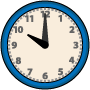Example 1: A school bell rings every half-hour. If it just rang, then how many times will it ring in in the next three and one-half hours?

Analysis: This problem is asking: How many halves are in three and one-half?

Step 1: Let's use shapes to represent the mixed number three and one-half.Step 2:Solution:In example 1, we used shapes to help us solve the problem. Let's look at example 2.

Example 2: A school bell rings every half-hour. If it just rang, then how many times will it ring in in the next nine and one-half hours?

Analysis: Using shapes to solve this problem would not be practical. We need to find another method.

Recall that a mixed number consists of a whole-number part and a fractional part. For example: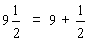Procedure: To write a mixed number as an improper fraction:

1. Write the whole-number part as an improper fraction, using the denominator from the fractional part.
2. Add the result from step 1 to the fractional part of the mixed number.

Let's use the procedure above to solve the problem from example 2.

Example 2: A school bell rings every half-hour.  If it just rang, then how many times will it ring in in the next nine and one-half hours?

Analysis: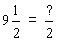Step 1: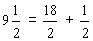Step 2:Solution: The school bell will ring 19 times in the next nine and one-half hours.

Let's look at some more examples of writing a mixed number as an improper fraction.

Example 3: Write two and three-fourths as an improper fraction.

Analysis: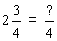Step 1:Step 2:Solution: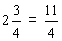Example 4: Write six and two-thirds as an improper fraction.

Analysis: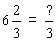Step 1: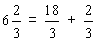Step 2: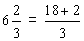Solution: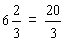Here is a summary of examples 1 to 4. Do you see a pattern? Do you see an easier way to write a mixed number as an improper fraction?

 Examples 1 to 4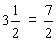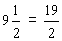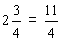There is a shortcut we can take for writing a mixed number as an improper fraction: If you multiply the denominator by the whole-number part, then add the numerator, the result gives you the numerator of the improper fraction. This is shown below for the mixed number two and three-fourths.Recapping examples 1 to 4, we get:

 Mixed Number Converted to an Improper Fraction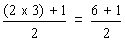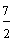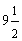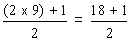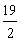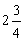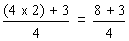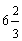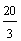Let's look at some more examples of writing a mixed number as an improper fraction using this shortcut.

Example 5: Write eleven and three-fifths as an improper fraction.

Analysis: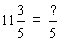Step 1: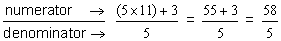Solution: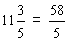Example 6: Write fourteen and one-third as an improper fraction.

Analysis: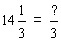Step 1: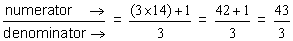Solution: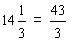This shortcut uses only one step, and makes it easier to convert large mixed numbers into improper fractions.

Summary: There are several methods for converting a mixed number into an improper fraction. Use the one that is appropriate for the given problem.

### Exercises

In Exercises 1 through 5, click once in an ANSWER BOX and type in your answer; then click ENTER. After you click ENTER, a message will appear in the RESULTS BOX to indicate whether your answer is correct or incorrect. To start over, click CLEAR. Note: To write the improper fraction five-thirds, enter 5/3 into the form.

 1. Write one and three-fourths as an improper fraction. ANSWER BOX:   RESULTS BOX:
 2. Write four and one-fifth as an improper fraction. ANSWER BOX:   RESULTS BOX:
 3. Write five and seven-eighths as an improper fraction. ANSWER BOX:   RESULTS BOX:
 4. Write fifteen and two-thirds as an improper fraction. ANSWER BOX:   RESULTS BOX:
 5. A recipe calls for two and three-fourths cups of milk. If the measuring cup holds only one-fourth cup, then how many times will you have to fill it? ANSWER BOX:  times   RESULTS BOX:
 Lessons on Fractions 1. Introduction to Fractions 2. Classifying Fractions 3. Equivalent Fractions 4. Simplifying Fractions 5. Comparing Fractions 6. Ordering Fractions 7. Converting Fractions to Mixed Numbers 8. Converting Mixed Numbers to Fractions 9. Practice Exercises 10. Challenge Exercises 11. Solutions